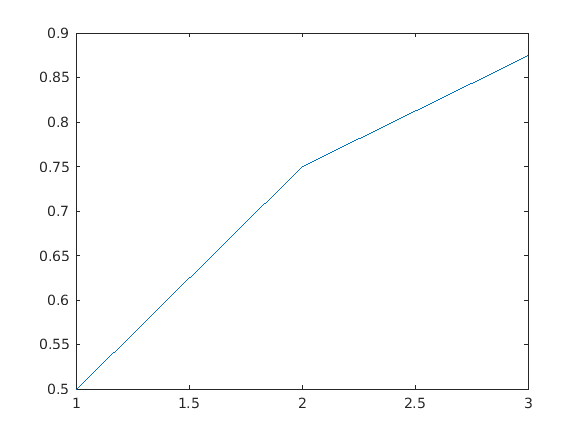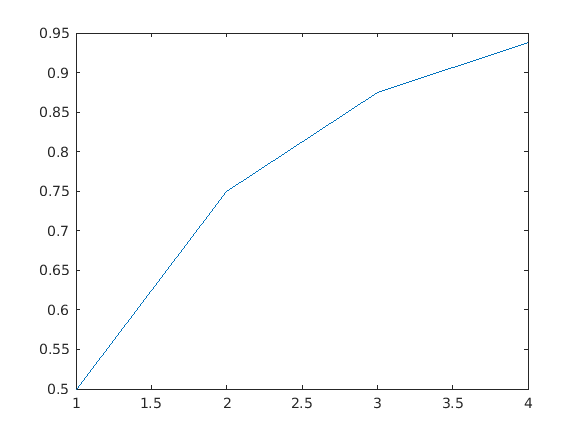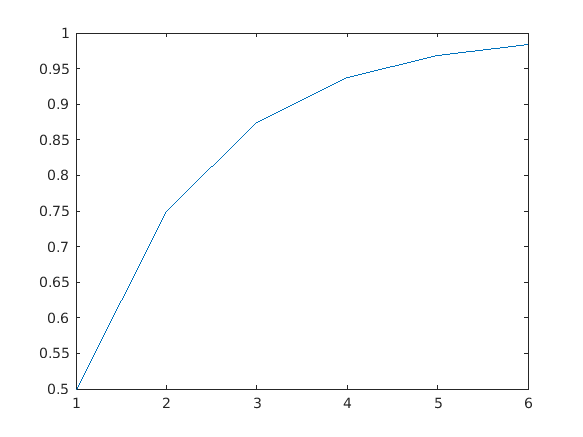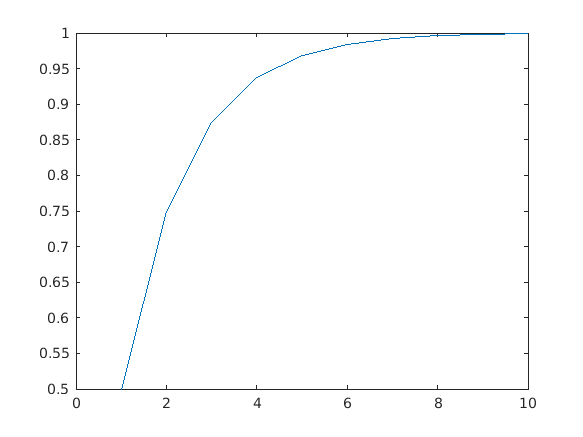Cody

# Problem 45248. Aquiles y la tortuga

Solution 2098317

Submitted on 19 Jan 2020 by Arnau Garcia
This solution is locked. To view this solution, you need to provide a solution of the same size or smaller.

### Test Suite

Test Status Code Input and Output
1   Pass
n = 3; suma = [1/2 (1/2+1/4) (1/2+1/4+1/8)]; assert(isequal(mi_funcion(n),suma));

``
2   Pass
n = 4; suma = [1/2 (1/2+1/4) (1/2+1/4+1/8) (1/2+1/4+1/8+1/16)]; assert(isequal(mi_funcion(n),suma))

``
3   Pass
n = 6; suma = [1/2 (1/2+1/4) (1/2+1/4+1/8) (1/2+1/4+1/8+1/16) (1/2+1/4+1/8+1/16+1/32) (1/2+1/4+1/8+1/16+1/32+1/64)]; assert(isequal(mi_funcion(n),suma))

``
4   Pass
n = 10; suma = [1/2 (1/2+1/4) (1/2+1/4+1/8) (1/2+1/4+1/8+1/16) (1/2+1/4+1/8+1/16+1/32) (1/2+1/4+1/8+1/16+1/32+1/64) (1/2+1/4+1/8+1/16+1/32+1/64+1/2^7) (1/2+1/4+1/8+1/16+1/32+1/64+1/2^7+1/2^8) (1/2+1/4+1/8+1/16+1/32+1/64+1/2^7+1/2^8+1/2^9) (1/2+1/4+1/8+1/16+1/32+1/64+1/2^7+1/2^8+1/2^9+1/2^10)]; assert(isequal(mi_funcion(n),suma))

``

### Community Treasure Hunt

Find the treasures in MATLAB Central and discover how the community can help you!

Start Hunting!